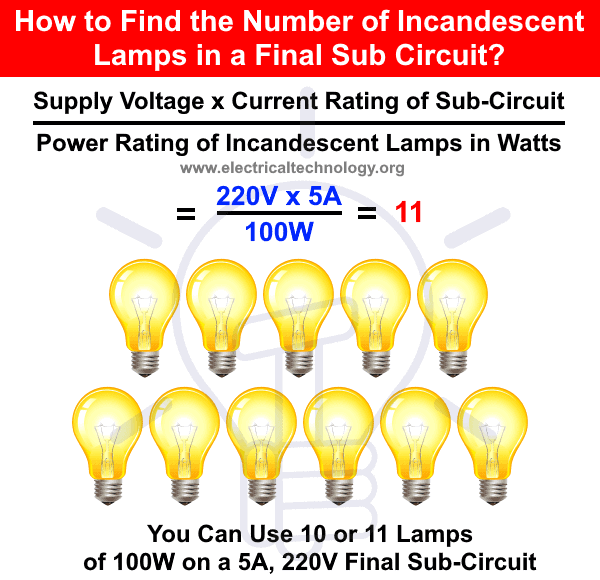# How to Calculate the Number of Incandescent Lamps in a Final Sub Circuit?

## How to Determine the Number of Incandescent Lamps in a Final Sub Circuit?

If the current rating of a final sub circuit is decided before and proper cable and wire size has been installed according to the rating and we want to determine that how many light points should be there at final sub-circuit. The following formula can be used to find the number of light and bulbs in the final sub circuit.

The power rating of light bulbs are different as there are different power rating bulbs are available in the market according to your needs.

Suppose, we have to install 100 Watts light bulbs in home, office or hall etc for normal lighting and the current rating of the specific final sub-circuit is 5 Amperes with supply voltage of 220V (110-120V in US). The maximum numbers of lamps can be calculated by the following formula.

Supply Power in Watts (V x I) / Load Power in Watts (P)

= Supply Voltage x Current Rating of Sub-Circuit / Power Rating of Incandescent Lamps in Watts

= (220V x 5A) / 100W

= 11

It shows, you can use 11 numbers of incandescent lamps each of having 100W on that 5A, 220V final sub circuit.Keep in mind that, it is not recommended to use more than 10 incandescent lamps on a final sub circuit for normal lighting. The high power rating should be used to calculate the number of incandescent lamps in a final sun circuit In case of special bulbs with high power rating.

Suppose, we have to find the number of incandescent lamps in a final sub circuit having a 10A and 120V AC supply.

120V x 10A / 150W

= 8

In this case, the maximum number of incandescent lamps in the final sub circuit is 8.

Note:

• Final sub-circuits are designed for max demand i.e. it consumes max power while appliances are in use.
• Power factor has been consider as unity due to resistive load in incandescent lamps.

Related Posts:

### Electrical Technology

1. Anonymous says:

If we want to install both kind of lamps(florescent and incandescent) then what is the formula? and in case of florescent lamp why we multiply the ratings of lamps by 2??? plz feed back me at live007messenger@mail.com thanx in advance

1. If you want to install both kind of lamps… Simply use
(Supply voltage * current rating of the sub circuit) / ((2* power of fluorescent lamps)+ power of incandescent lamps)
i.e. use the highest power rating.

2. Rana says:

How many are Fluorescent lamps incandescent lamps?

1. These are the minimum numbers of the different type of lamps which has to be installed in the final sub circuit…. There is another post for finding the number of fluorescent lamps and tube lights. In case of mix lighting points and bulbs with different wattage rating, use the highest power rating for division to be safe.

3. Shams says:

Please can some one send a detailed wiring diagram of ring mains circuit for lighting points for me. Not power ring circuit but lighting ring mains.